# Logic Gates | Definitions | Types | Symbols | Truth Tables

## Logic Gates-

Logic Gates may be defined as-

 Logic gates are the digital circuits capable of performing a particular logic function by operating on a number of binary inputs. OR Logic gates are the basic building blocks of any digital circuit.

## Types Of Logic Gates-

Logic gates can be broadly classified as-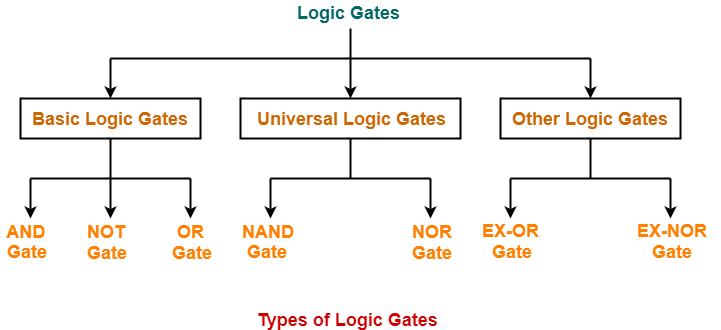## Basic Logic Gates-

Basic Logic Gates are the fundamental logic gates using which universal logic gates and other logic gates are constructed.

They have the following properties-

• Basic logic gates are associative in nature.
• Basic logic gates are commutative in nature.

There are following three basic logic gates-

1. AND Gate
2. OR Gate
3. NOT Gate

## 1. AND Gate-

• The output of AND gate is high (‘1’) if all of its inputs are high (‘1’).
• The output of AND gate is low (‘0’) if any one of its inputs is low (‘0’).

### Logic Symbol-

The logic symbol for AND Gate is as shown below-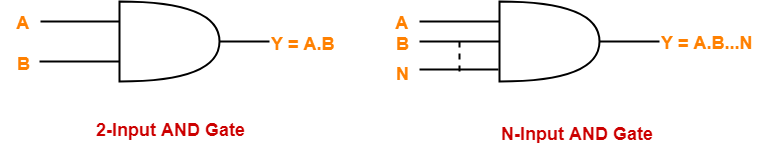### Truth Table-

The truth table for AND Gate is as shown below-

 A B Y = A.B 0 0 0 0 1 0 1 0 0 1 1 1

### Timing Diagram-

The timing diagram for AND Gate is as shown below-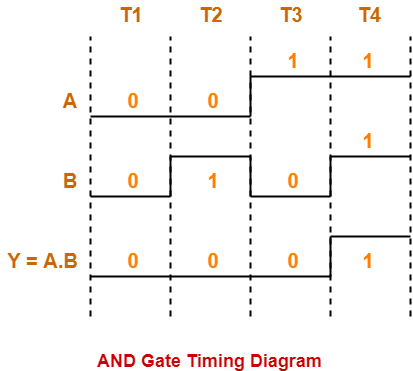## 2. OR Gate-

• The output of OR gate is high (‘1’) if any one of its inputs is high (‘1’).
• The output of OR gate is low (‘0’) if all of its inputs are low (‘0’).

### Logic Symbol-

The logic symbol for OR Gate is as shown below-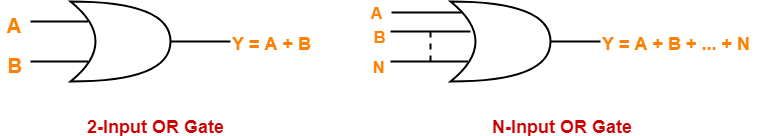### Truth Table-

The truth table for OR Gate is as shown below-

 A B Y = A + B 0 0 0 0 1 1 1 0 1 1 1 1

### Timing Diagram-

The timing diagram for OR Gate is as shown below-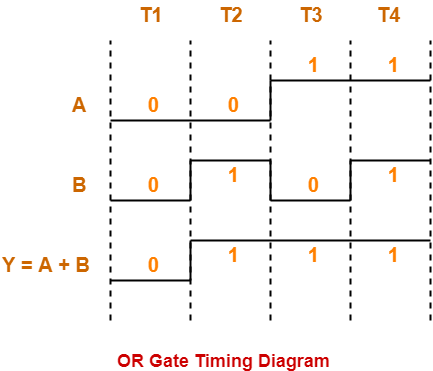## 3. NOT Gate-

• The output of NOT gate is high (‘1’) if its input is low (‘0’).
• The output of NOT gate is low (‘0’) if its input is high (‘1’).

From here-

• It is clear that NOT gate simply inverts the given input.
• Since NOT gate simply inverts the given input, therefore it is also known as Inverter Gate.

### Logic Symbol-

The logic symbol for NOT Gate is as shown below-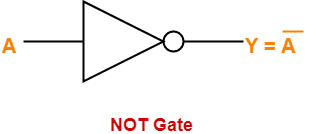### Truth Table-

The truth table for NOT Gate is as shown below-

 A Y = A’ 0 1 1 0

### Timing Diagram-

The timing diagram for NOT Gate is as shown below-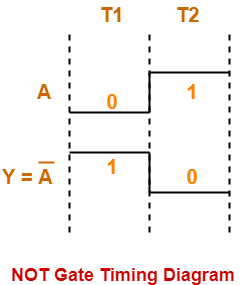To gain better understanding about Logic Gates,

Watch this Video Lecture

Next Article- Universal Logic Gates

Get more notes and other study material of Digital Design.

Watch video lectures by visiting our YouTube channel LearnVidFun.

SummaryArticle Name
Logic Gates | Definitions | Types | Symbols | Truth Tables
Description
Logic gates are defined as the basic building blocks of any digital circuit. Logic Gates Definitions, Types, Symbols and Truth Tables are discussed. There are 3 basic logic gates- AND Gate, OR Gate, NOT Gate.
Author
Publisher Name
Gate Vidyalay
Publisher Logo